Kubrnete191 1 0

## 初步认识动态规划

• 通过在每个局部的问题中都求出最优解，从而得到全局的最优解：对于贪心算法而言，通过一系列局部最优的选择得到所求问题的整体最优解。其中选择的贪心策略必须具备无后效性(某个状态以前的过程不会影响以后的状态，只与当前状态有关)。当前最优解从子问题最优解即可得出，即由上一步的最优解推导下一步的最优解，而上一部之前的最优解则不作保留。并且，每一步的最优解一定包含上一步的最优解。
• 通过求出的局部最优解来推导全局最优解: 对于动态规划算法而言，全局最优解中一定包含某个局部最优解，但不一定包含前一个局部最优解，因此需要记录之前的所有最优解。将待求解的问题分解为若干个子问题（阶段），通过求解前一子问题(阶段)的解为后一子问题的求解提供了有用的信息。在求解任一子问题时，通过决策保留那些有可能达到最优的局部解。依次解决各子问题，最后一个子问题就是初始问题的解。

## 与动态规划有关的理论知识：

1. 最优性原理
2. 无后效性
3. 有重叠子问题(非必要性质)

int V_total(int i, int j)
{
if (values[i][j])
return values[i][j]; //如果结果已经计算过，直接返回
int value = 0;
if (i == 0 || j == 0) //当可选物品数量或背包容量为0时，返回0
value = 0;
else if (j < w[i]) //背包容量不足
value = V_total(i - 1, j);
else
{
value = max(V_total(i - 1, j), V_total(i - 1, j - w[i]) + v[i]);
values[i][j] = value;
}
return value;
}（提交仍TLE）

## 动态规划中的最优决策表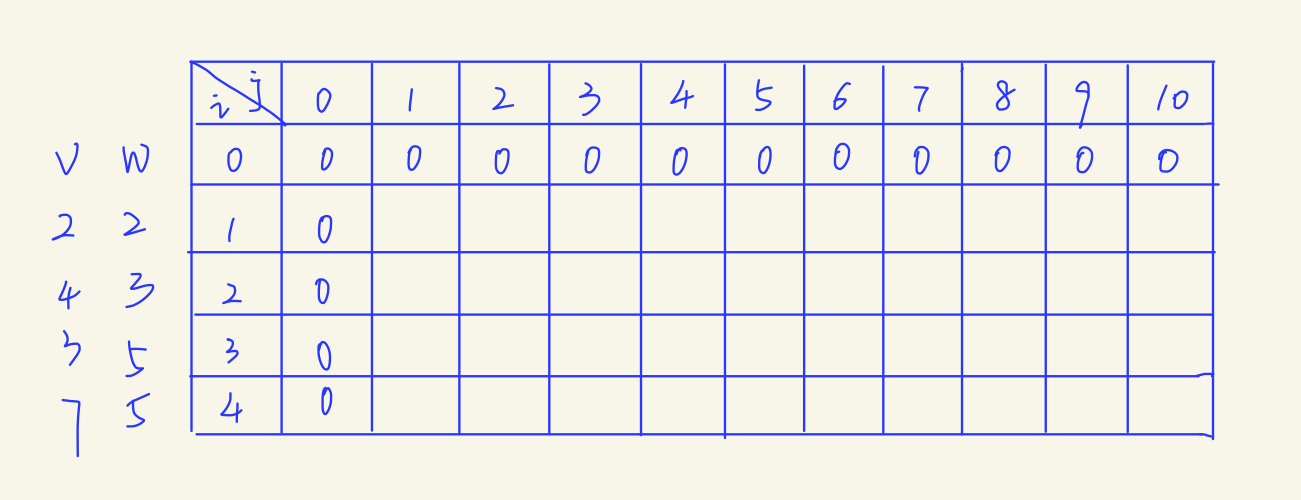)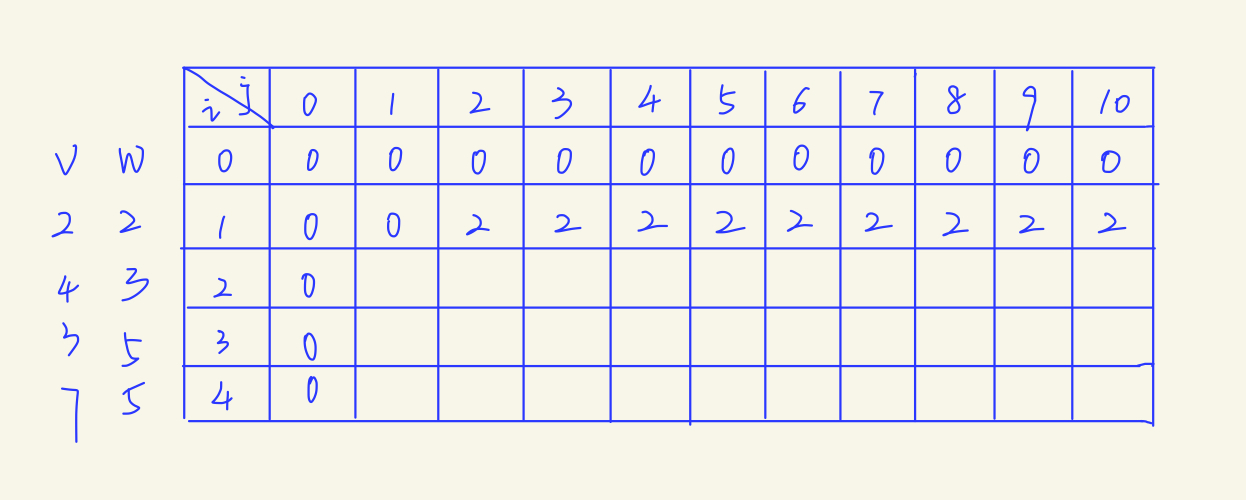)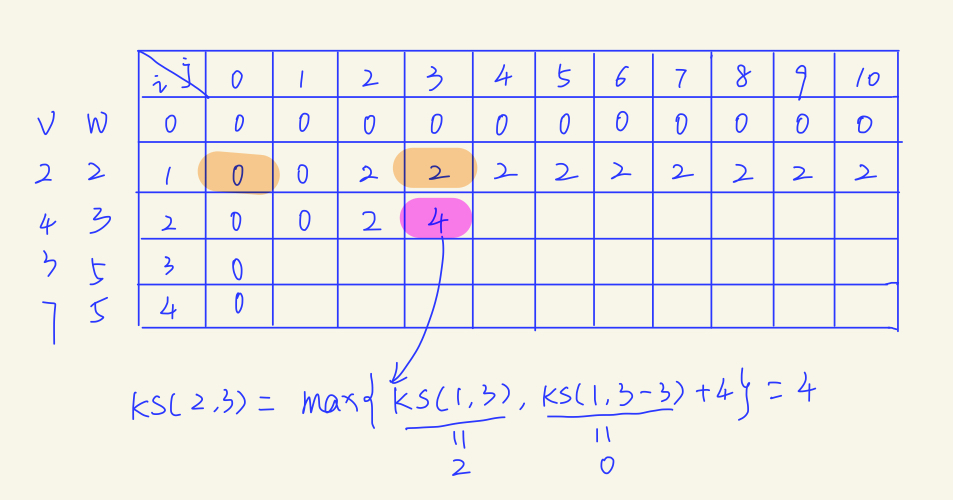)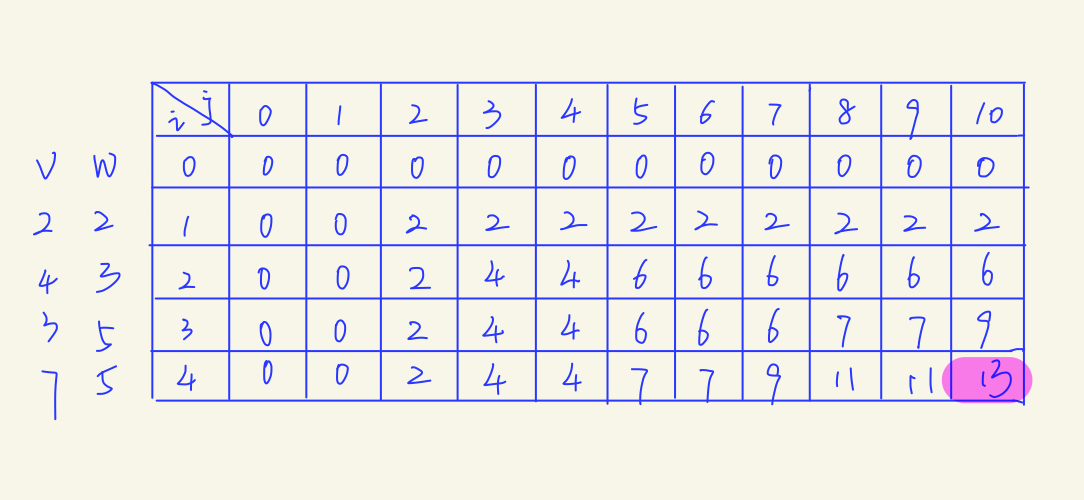)for (int i = 1; i <= n; i++) //开始填表，表中初始值为0
for (int j = 1; j <= c; j++)
if (j < w[i]) //背包容量不够
values[i][j] = values[i - 1][j];
else //将价值最优的填入表中
values[i][j] = max(values[i - 1][j], values[i - 1][j - w[i]] + v[i]);
cout << values[i][j];(终于我们将时间复杂度优化为填表时间O(nc)，然而空间复杂度也是O(nc),于是不可避免地MLE了...看来还不能收工，让我们继续优化，坚持住！)

## 最终版动态规划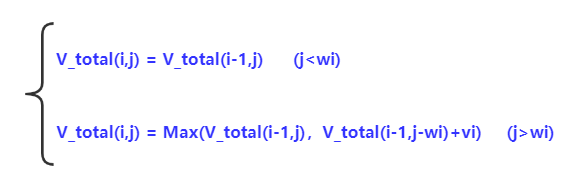)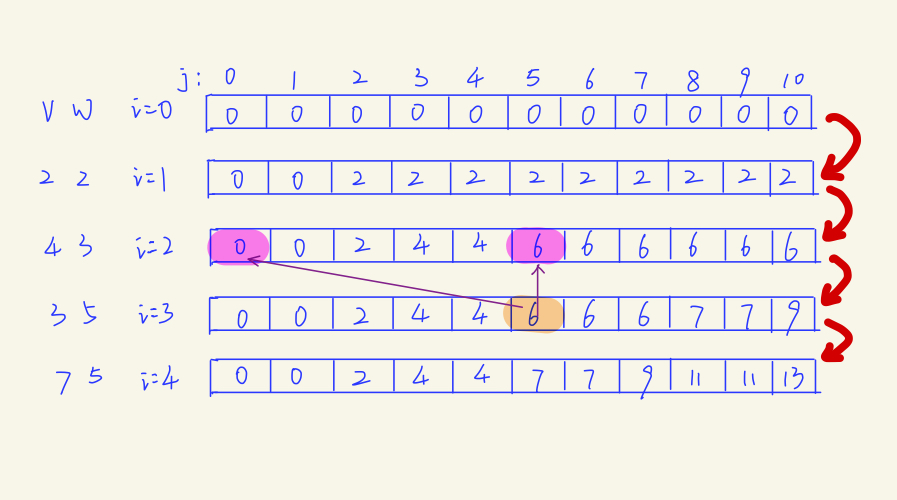)V_total(j) = max{V_total(j),V_total(j - wi) + vi}

(如果不理解，尝试通过将状态转移方程与上面的递推公式对比。每次求解 V_total(i,j)只与V_total(i-1,j or j-wi) 有关)

V_total(8) = max{V_total(8),V_total(8 - 5) + 3} = 7。在这里计算V_total(8)的时候，我们就把原来V_total(8)的内容修改掉了。这样，我们后续计算就无法找到这个位置的原值，也就是上一轮循环中计算出来的值了。

#include <bits/stdc++.h>
using namespace std;
int n, c, d;
int v, w, dp;
int main()
{

cin >> n >> c >> d;
for (int i = 1; i <= n; i++)
cin >> w[i] >> v[i];
for (int i = 1; i <= n; i++) //遍历每个物品，开始填表
for (int j = c; j >= w[i];j--) //从后往前，倒序推导
dp[j] = max(dp[j], dp[j - w[i]] + v[i]);
cout << d + dp[c]; //返回最右下角的格子的值即问题的解
}### 总结：

• 动态规划问题，大致可以通过以下四部进行解决。

1.划分状态，即划分子问题(核心思想）

2.状态表示，即如何让计算机理解子问题。(用二维数组表示最优决策表中每个子问题的解）

3.状态转移，即父问题是如何由子问题推导出来的(核心步骤，通过递推关系、问题的阶段和每个阶段的状态推导状态转移方程)。

4.确定边界，确定初始状态是什么、最小的子问题以及最终状态。(最后，开始写代码吧！)Python正则表达式 2021-03-13 17:43